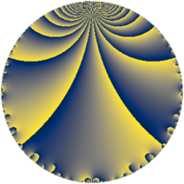# Properties

 Label 38.3.bLevel $38$ Weight $3$ Character orbit 38.b Rep. character $\chi_{38}(37,\cdot)$ Character field $\Q$ Dimension $2$ Newform subspaces $1$ Sturm bound $15$ Trace bound $0$

# Related objects

## Defining parameters

 Level: $$N$$ $$=$$ $$38 = 2 \cdot 19$$ Weight: $$k$$ $$=$$ $$3$$ Character orbit: $$[\chi]$$ $$=$$ 38.b (of order $$2$$ and degree $$1$$) Character conductor: $$\operatorname{cond}(\chi)$$ $$=$$ $$19$$ Character field: $$\Q$$ Newform subspaces: $$1$$ Sturm bound: $$15$$ Trace bound: $$0$$

## Dimensions

The following table gives the dimensions of various subspaces of $$M_{3}(38, [\chi])$$.

Total New Old
Modular forms 12 2 10
Cusp forms 8 2 6
Eisenstein series 4 0 4

## Trace form

 $$2 q - 4 q^{4} - 2 q^{5} - 8 q^{6} + 10 q^{7} + 2 q^{9} + O(q^{10})$$ $$2 q - 4 q^{4} - 2 q^{5} - 8 q^{6} + 10 q^{7} + 2 q^{9} + 10 q^{11} + 8 q^{16} - 50 q^{17} + 38 q^{19} + 4 q^{20} - 20 q^{23} + 16 q^{24} - 48 q^{25} + 48 q^{26} - 20 q^{28} + 8 q^{30} - 10 q^{35} - 4 q^{36} + 96 q^{39} - 40 q^{42} + 10 q^{43} - 20 q^{44} - 2 q^{45} + 10 q^{47} - 48 q^{49} - 80 q^{54} - 10 q^{55} - 120 q^{58} + 190 q^{61} + 120 q^{62} + 10 q^{63} - 16 q^{64} - 40 q^{66} + 100 q^{68} - 50 q^{73} + 72 q^{74} - 76 q^{76} + 50 q^{77} - 8 q^{80} - 142 q^{81} + 120 q^{82} - 260 q^{83} + 50 q^{85} - 240 q^{87} + 40 q^{92} + 240 q^{93} - 38 q^{95} - 32 q^{96} + 10 q^{99} + O(q^{100})$$

## Decomposition of $$S_{3}^{\mathrm{new}}(38, [\chi])$$ into newform subspaces

Label Dim $A$ Field CM Traces $q$-expansion
$a_{2}$ $a_{3}$ $a_{5}$ $a_{7}$
38.3.b.a $2$ $1.035$ $$\Q(\sqrt{-2})$$ None $$0$$ $$0$$ $$-2$$ $$10$$ $$q+\beta q^{2}+2\beta q^{3}-2q^{4}-q^{5}-4q^{6}+\cdots$$

## Decomposition of $$S_{3}^{\mathrm{old}}(38, [\chi])$$ into lower level spaces

$$S_{3}^{\mathrm{old}}(38, [\chi]) \cong$$ $$S_{3}^{\mathrm{new}}(19, [\chi])$$$$^{\oplus 2}$$